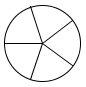### Home > MC1 > Chapter 6 > Lesson 6.1.2 > Problem6-16

6-16.

1. How many fifths are there in a whole?2. How many thirds are there in $2\frac{1}{3}$?

$\text{There are } \frac{3}{3}\text{ in 1. Use this information to help you.}$

3. How many $\frac { 2 } { 5 }$are there in $4$?

Use the picture below to help with this one.